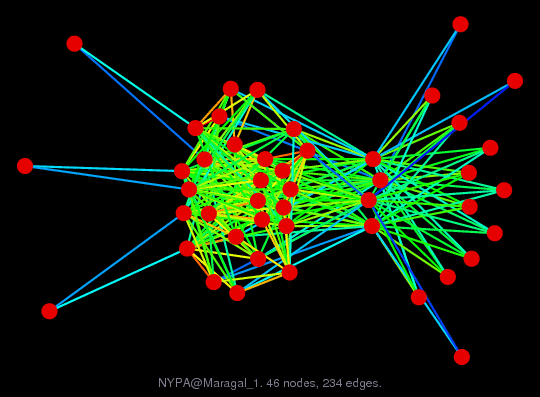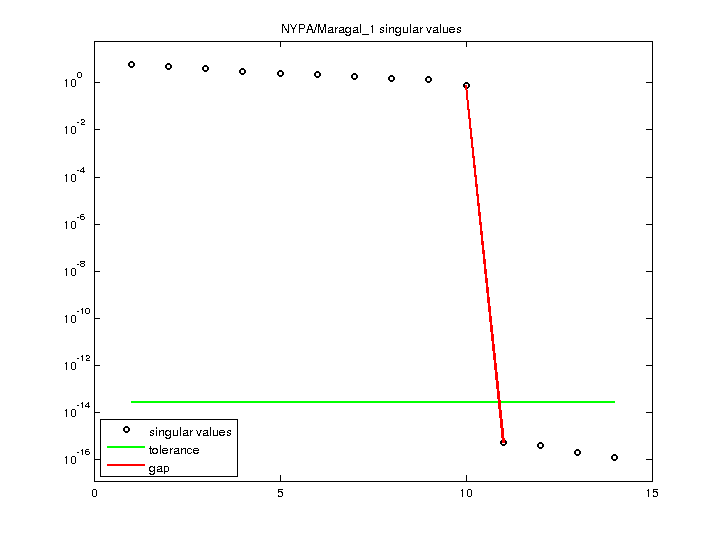Matrix: NYPA/Maragal_1

Description: Rank deficient least squares problem, D. Maragal, NY Power Authority(bipartite graph drawing)• Matrix group: NYPA
• download as a MATLAB mat-file, file size: 2 KB. Use UFget(1884) or UFget('NYPA/Maragal_1') in MATLAB.

 Matrix properties number of rows 32 number of columns 14 nonzeros 234 structural full rank? yes structural rank 14 # of blocks from dmperm 1 # strongly connected comp. 1 explicit zero entries 0 nonzero pattern symmetry 0% numeric value symmetry 0% type real structure rectangular Cholesky candidate? no positive definite? no

 author D. Maragal editor T. Davis date 2008 kind least squares problem 2D/3D problem? no

 Additional fields size and type b full 32-by-1

Notes:

```rank deficient (rank(A) < sprank(A) == size(A,2))
rank: 10 sprank: 14 columns: 14
```

 Ordering statistics: result nnz(V) for QR, upper bound nnz(L) for LU, with COLAMD 329 nnz(R) for QR, upper bound nnz(U) for LU, with COLAMD 105

 SVD-based statistics: norm(A) 5.93273 min(svd(A)) 1.27953e-16 cond(A) 4.63664e+16 rank(A) 10 sprank(A)-rank(A) 4 null space dimension 4 full numerical rank? no singular value gap 1.46088e+15

 singular values (MAT file): click here SVD method used: s = svd (full (R)) ; where [~,R,E] = spqr (A) with droptol of zero status: ok